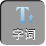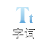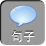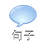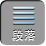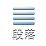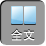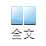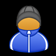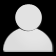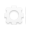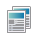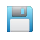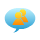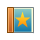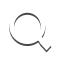-AA+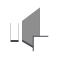“变”则 “通” ——与幂有关试题的解题技巧介绍

1、计算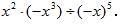2、如果 2 m+ 3 n -3= 0，求 9 m× 27 n.

3、已知 a= 81 31b= 27 41c= 9 61,则 abc的大小关系是（）.

(A) abc

(B) acb

(C) abc

(D) bca

4、计算（－ 0.125 2013× 8 2014

5、已知 a= 3 55b= 4 44c= 5 33，则 abc的大小关系是（）

(A) abc

(B) cba

(C) cab

(D) acb

6、已知 a 3 m= 3a 2 n= 2，求 a 6 m+ 4 n的值